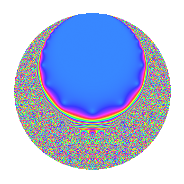Properties

 Label 8.16.a.cLevel 8 Weight 16 Character orbit 8.a Self dual Yes Analytic conductor 11.415 Analytic rank 0 Dimension 2 CM No Inner twists 1

Related objects

Newspace parameters

 Level: $$N$$ = $$8 = 2^{3}$$ Weight: $$k$$ = $$16$$ Character orbit: $$[\chi]$$ = 8.a (trivial)

Newform invariants

 Self dual: Yes Analytic conductor: $$11.415480408$$ Analytic rank: $$0$$ Dimension: $$2$$ Coefficient field: $$\Q(\sqrt{58})$$ Coefficient ring: $$\Z[a_1, a_2, a_3]$$ Coefficient ring index: $$2^{7}\cdot 5$$ Fricke sign: $$1$$ Sato-Tate group: $\mathrm{SU}(2)$

$q$-expansion

Coefficients of the $$q$$-expansion are expressed in terms of $$\beta = 640\sqrt{58}$$. We also show the integral $$q$$-expansion of the trace form.

 $$f(q)$$ $$=$$ $$q$$ $$+ ( -2036 + \beta ) q^{3}$$ $$+ ( -70130 + 36 \beta ) q^{5}$$ $$+ ( 63096 + 90 \beta ) q^{7}$$ $$+ ( 13553189 - 4072 \beta ) q^{9}$$ $$+O(q^{10})$$ $$q$$ $$+ ( -2036 + \beta ) q^{3}$$ $$+ ( -70130 + 36 \beta ) q^{5}$$ $$+ ( 63096 + 90 \beta ) q^{7}$$ $$+ ( 13553189 - 4072 \beta ) q^{9}$$ $$+ ( 10341316 + 17307 \beta ) q^{11}$$ $$+ ( 249903238 + 2340 \beta ) q^{13}$$ $$+ ( 998029480 - 143426 \beta ) q^{15}$$ $$+ ( 1569758450 + 175896 \beta ) q^{17}$$ $$+ ( -237334276 + 285813 \beta ) q^{19}$$ $$+ ( 2009648544 - 120144 \beta ) q^{21}$$ $$+ ( -20388001304 - 407250 \beta ) q^{23}$$ $$+ ( 5189451575 - 5049360 \beta ) q^{25}$$ $$+ ( -95117607752 + 7494874 \beta ) q^{27}$$ $$+ ( 17626578678 + 19501524 \beta ) q^{29}$$ $$+ ( -17194596640 - 30813336 \beta ) q^{31}$$ $$+ ( 390104018224 - 24895736 \beta ) q^{33}$$ $$+ ( 72547109520 - 4040244 \beta ) q^{35}$$ $$+ ( 435114282222 + 65307348 \beta ) q^{37}$$ $$+ ( -453212080568 + 245138998 \beta ) q^{39}$$ $$+ ( 450042726042 - 384323472 \beta ) q^{41}$$ $$+ ( 250353999268 - 376557597 \beta ) q^{43}$$ $$+ ( -4433041970170 + 773484164 \beta ) q^{45}$$ $$+ ( 604029559632 - 356149188 \beta ) q^{47}$$ $$+ ( -4551150324727 + 11357280 \beta ) q^{49}$$ $$+ ( 982697888600 + 1211634194 \beta ) q^{51}$$ $$+ ( 618367101022 - 1167361452 \beta ) q^{53}$$ $$+ ( 14076485262520 - 841452534 \beta ) q^{55}$$ $$+ ( 7273214864336 - 819249544 \beta ) q^{57}$$ $$+ ( 7220987952532 + 884641527 \beta ) q^{59}$$ $$+ ( 9168151708630 + 1334372868 \beta ) q^{61}$$ $$+ ( -7851240050856 + 962860098 \beta ) q^{63}$$ $$+ ( -15524441248940 + 8832412368 \beta ) q^{65}$$ $$+ ( -38300710757428 - 8202370383 \beta ) q^{67}$$ $$+ ( 31835013854944 - 19558840304 \beta ) q^{69}$$ $$+ ( -72938586943432 + 1679200362 \beta ) q^{71}$$ $$+ ( -13208634962054 + 17753485176 \beta ) q^{73}$$ $$+ ( -130522359054700 + 15469948535 \beta ) q^{75}$$ $$+ ( 37656800058336 + 2022720912 \beta ) q^{77}$$ $$+ ( 128953916694064 + 12009529764 \beta ) q^{79}$$ $$+ ( 177240223511849 - 51948421912 \beta ) q^{81}$$ $$+ ( 127756403291324 - 35231817627 \beta ) q^{83}$$ $$+ ( 40346979242300 + 44175717720 \beta ) q^{85}$$ $$+ ( 427406091174792 - 22078524186 \beta ) q^{87}$$ $$+ ( -359897305856406 + 61727093496 \beta ) q^{89}$$ $$+ ( 20771076784848 + 22638936060 \beta ) q^{91}$$ $$+ ( -697018061925760 + 45541355456 \beta ) q^{93}$$ $$+ ( 261084334798280 - 28588099626 \beta ) q^{95}$$ $$+ ( 203817795209378 - 259618417416 \beta ) q^{97}$$ $$+ ( -1534081383650476 + 192455203271 \beta ) q^{99}$$ $$+O(q^{100})$$ $$\operatorname{Tr}(f)(q)$$ $$=$$ $$2q$$ $$\mathstrut -\mathstrut 4072q^{3}$$ $$\mathstrut -\mathstrut 140260q^{5}$$ $$\mathstrut +\mathstrut 126192q^{7}$$ $$\mathstrut +\mathstrut 27106378q^{9}$$ $$\mathstrut +\mathstrut O(q^{10})$$ $$2q$$ $$\mathstrut -\mathstrut 4072q^{3}$$ $$\mathstrut -\mathstrut 140260q^{5}$$ $$\mathstrut +\mathstrut 126192q^{7}$$ $$\mathstrut +\mathstrut 27106378q^{9}$$ $$\mathstrut +\mathstrut 20682632q^{11}$$ $$\mathstrut +\mathstrut 499806476q^{13}$$ $$\mathstrut +\mathstrut 1996058960q^{15}$$ $$\mathstrut +\mathstrut 3139516900q^{17}$$ $$\mathstrut -\mathstrut 474668552q^{19}$$ $$\mathstrut +\mathstrut 4019297088q^{21}$$ $$\mathstrut -\mathstrut 40776002608q^{23}$$ $$\mathstrut +\mathstrut 10378903150q^{25}$$ $$\mathstrut -\mathstrut 190235215504q^{27}$$ $$\mathstrut +\mathstrut 35253157356q^{29}$$ $$\mathstrut -\mathstrut 34389193280q^{31}$$ $$\mathstrut +\mathstrut 780208036448q^{33}$$ $$\mathstrut +\mathstrut 145094219040q^{35}$$ $$\mathstrut +\mathstrut 870228564444q^{37}$$ $$\mathstrut -\mathstrut 906424161136q^{39}$$ $$\mathstrut +\mathstrut 900085452084q^{41}$$ $$\mathstrut +\mathstrut 500707998536q^{43}$$ $$\mathstrut -\mathstrut 8866083940340q^{45}$$ $$\mathstrut +\mathstrut 1208059119264q^{47}$$ $$\mathstrut -\mathstrut 9102300649454q^{49}$$ $$\mathstrut +\mathstrut 1965395777200q^{51}$$ $$\mathstrut +\mathstrut 1236734202044q^{53}$$ $$\mathstrut +\mathstrut 28152970525040q^{55}$$ $$\mathstrut +\mathstrut 14546429728672q^{57}$$ $$\mathstrut +\mathstrut 14441975905064q^{59}$$ $$\mathstrut +\mathstrut 18336303417260q^{61}$$ $$\mathstrut -\mathstrut 15702480101712q^{63}$$ $$\mathstrut -\mathstrut 31048882497880q^{65}$$ $$\mathstrut -\mathstrut 76601421514856q^{67}$$ $$\mathstrut +\mathstrut 63670027709888q^{69}$$ $$\mathstrut -\mathstrut 145877173886864q^{71}$$ $$\mathstrut -\mathstrut 26417269924108q^{73}$$ $$\mathstrut -\mathstrut 261044718109400q^{75}$$ $$\mathstrut +\mathstrut 75313600116672q^{77}$$ $$\mathstrut +\mathstrut 257907833388128q^{79}$$ $$\mathstrut +\mathstrut 354480447023698q^{81}$$ $$\mathstrut +\mathstrut 255512806582648q^{83}$$ $$\mathstrut +\mathstrut 80693958484600q^{85}$$ $$\mathstrut +\mathstrut 854812182349584q^{87}$$ $$\mathstrut -\mathstrut 719794611712812q^{89}$$ $$\mathstrut +\mathstrut 41542153569696q^{91}$$ $$\mathstrut -\mathstrut 1394036123851520q^{93}$$ $$\mathstrut +\mathstrut 522168669596560q^{95}$$ $$\mathstrut +\mathstrut 407635590418756q^{97}$$ $$\mathstrut -\mathstrut 3068162767300952q^{99}$$ $$\mathstrut +\mathstrut O(q^{100})$$

Embeddings

For each embedding $$\iota_m$$ of the coefficient field, the values $$\iota_m(a_n)$$ are shown below.

For more information on an embedded modular form you can click on its label.

Label $$\iota_m(\nu)$$ $$a_{2}$$ $$a_{3}$$ $$a_{4}$$ $$a_{5}$$ $$a_{6}$$ $$a_{7}$$ $$a_{8}$$ $$a_{9}$$ $$a_{10}$$
1.1
 −7.61577 7.61577
0 −6910.09 0 −245597. 0 −375573. 0 3.34005e7 0
1.2 0 2838.09 0 105337. 0 501765. 0 −6.29412e6 0
 $$n$$: e.g. 2-40 or 990-1000 Significant digits: Format: Complex embeddings Normalized embeddings Satake parameters Satake angles

Inner twists

This newform does not admit any (nontrivial) inner twists.

Atkin-Lehner signs

$$p$$ Sign
$$2$$ $$1$$

Hecke kernels

This newform can be constructed as the kernel of the linear operator $$T_{3}^{2}$$ $$\mathstrut +\mathstrut 4072 T_{3}$$ $$\mathstrut -\mathstrut 19611504$$ acting on $$S_{16}^{\mathrm{new}}(\Gamma_0(8))$$.